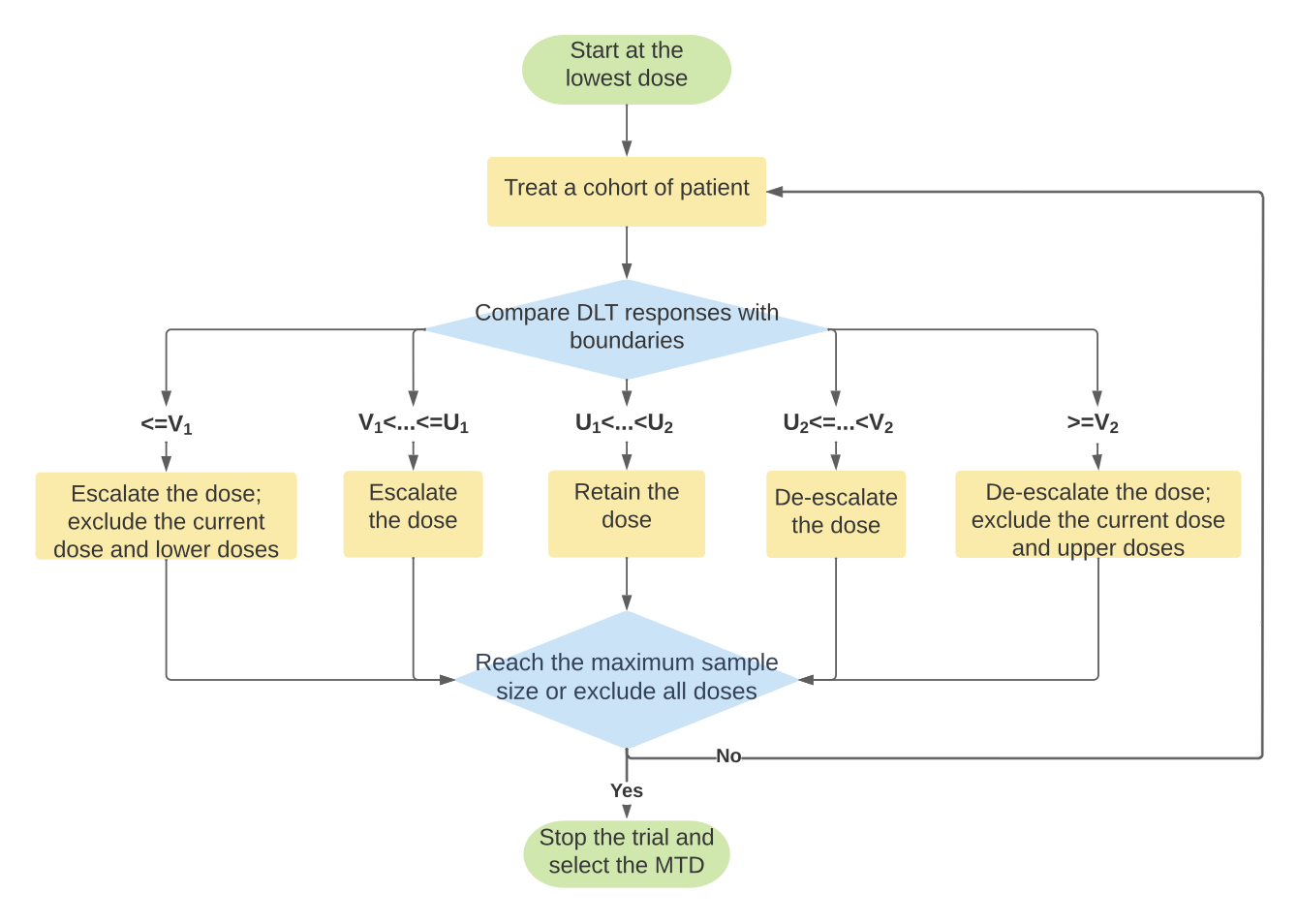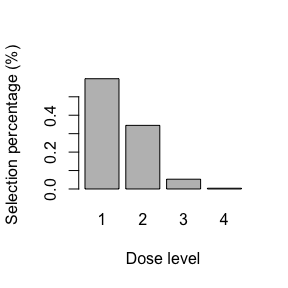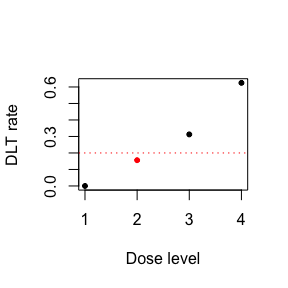# PoP Design

## Introduction

Model-assisted designs are cutting-edge adaptive designs to find the maximum tolerated dose (MTD) in phase I clinical trials. They enjoy superior performance comparable to more complicated, model-based adaptive designs, but with their decision rule pretabulated, they can be implemented as simply as the conventional algorithmic designs. In this work, we propose the posterior predictive (PoP) design, a novel model-assisted design to exploit Bayesian interval hypothesis testing, and develop a freely accessible R package PoPdesign to better facilitate the trial design application. Our work moves beyond the previous model-assisted designs by theoretically achieving consistency in selecting the true MTD and global optimality in dose transition. The simulation studies demonstrate that the PoP design can achieve significant improvement in operating characteristics to identify the MTD. Therefore, the PoP design provides a useful and convenient upgrade to the prevalent model-assisted designs.

## Methods

We assume that there are $$J$$ pre-specified dose levels of the drug of interest. Let $$d_1,d_2,\ldots,d_J$$ denote these dose levels. The dose-limiting toxicity (DLT) is assessed as a binary outcome, experiencing toxicity or not. The true dose toxicity is monotonically increasing as the dose level increases. Let $$\phi$$ be the target toxicity rate and $$\pi_j$$ be the true dose-toxicity of dose level $$d_j$$, for $$j=1,2,\ldots,J$$.

We formulate our hypothesis as:

$H_{0j}: \pi_j=\phi \\ H_{1j}: \pi_j\ne\phi$ $$H_{0j}$$ indicates that $$d_j$$ is the desired MTD so that we should stay; $$H_{1j}$$ reflects the current dose is either below or above the MTD so that we should transit to a lower or upper dose level. If the observed toxicity rate is above the target toxicity rate $$\phi$$, we de-escalate the dose; if the observed toxicity rate is below $$\phi$$, we escalate the dose.

Instead of the Bayes factor, we use the predictive Bayes factor (PrBF) (Zhou, 2011) to conduct the hypothesis testing. Denote $$M_k (\mathbf{y}),k=0,1$$ the posterior model under hypotheses $$H_k$$ with the parameter updated in the presence of observed data $$\mathbf{y}=\{y_1,y_2,\cdots,y_n\}$$. Denote $$\theta_k$$ the parameters considered in the model $$M_k$$ and specify a prior distribution as $$\pi(\theta_k)$$. The PrBF comparing $$H_0$$ to $$H_1$$ is defined as:

$\text{PrBF}_{1,2}=\frac{\prod_i P(y_i|M_1(\mathbf{y}))}{\prod_i P(y_i|M_2(\mathbf{y}))}\frac{\exp{(n\cdot \hat{b}_1})}{\exp{(n\cdot\hat{b}_2)}}$ where the estimator $$\hat{b}_k$$ corrects the over-estimation bias in the empirical log posterior predictive distribution, owing to the ‘double use’ of observed data in both model fitting and evaluation.

The PrBF has attractive features going beyond Bayes factors by employing the posterior predictive distribution in model assessment. It significantly reduces the sensitivity to the choice of the prior distribution and avoids the degeneration of the integrated likelihood underlying Lindley’s paradox.

We then use the PrBF to perform the hypothesis testing of the PoP design, We denote $$y_j$$ the sum of DLTs among $$n_j$$ subjects that received dose $$d_j$$, for $$j=1,2,\dots,J$$, following a binomial distribution:

$y_j\sim\text{Bin}(n_j,\pi_j)$

We apply the predictive Bayes factor (prBF) for dose transition.

### Dose transition

If $$\text{PrBF}_{0,1}<C(\phi,n_j)$$, the evidence is in favor of $$H_{1j}$$. We are going to transit the current dose. We assign the next cohort of patients to an adjacent dose according to $$y_j$$, such as

• If $$y_j<\phi \cdot n_j$$, we escalate the dose;

• If $$y_j>\phi \cdot n_j$$, we deescalate the dose.

Otherwise, we retain the current dose.

The threshold $$C(\phi, n_j)$$ describes the tolerance in strength of evidence for dose transition. Mathematically, it can be intrinsically determined by the loss function in making incorrect decisions.

### Dose elimination

For patient safety and trial efficiency, the PoP design employs a dose elimination rule. If the PrBF based on the observed $$y_j$$ indicates a dose is above the MTD with a certain evidence, we exclude the current dose and doses above to avoid treating patients at overly toxic doses; alternatively, if the PrBF implies that a dose is substantially below the MTD, we eliminate the current dose and doses below to prevent patients from receiving subtherapeutic doses. Such a dose elimination rule is as follow:

If $$\text{PrBF}_{0,1}<E(\phi, n_j)$$, the evidence is $$\textit{strongly}$$ in favor of $$H_{1j}$$ and:

• If $$y_j<\phi \cdot n_j$$, the current dose is deemed as subtherapeutic and we exclude the current dose and lower doses;

• If $$y_j>\phi \cdot n_j$$, the current dose is overly toxic and we exclude the current dose and higher doses.

Once all the doses are eliminated from further investigation, the trial is terminated early. The selection of the threshold $$E(\phi, n_j)$$ is critical for the PoP design, because it ensures the safety of the patients and efficiency of the design by influencing the early termination rule. The plot() output of the get.boundary.pop results demonstrates the process of the PoP design to conduct a trial.

### Theoretical properties

Compared to other model-assisted design, PoPdesign possesses these three theoretical properties:

$$\textit{Theorem 1: Global optimality}$$:

The proposed dose transition rules for PoP design minimizes the risk of incorrect decision of dose assignment under the Bayesian decision framework.

$$\textit{Theorem 2: Long-term coherence}$$:

The probability of dose escalation (or de-escalation) is zero when the observed toxicity rate $$\hat{\pi}_j$$ at the current dose is higher (or lower) than the target toxicity rate $$\phi$$.

$$\textit{Theorem 3: Consistency}$$:

Dose allocation based on the escalation and de-escalation boundaries in the PoP design converges almost surely to dose level $$j^*$$ if $$\exists j^*$$, s.t. $$\pi_{j^*}=\phi$$.

$$\textit{Global optimality}$$: and $$\textit{Consistency}$$ are two unique properties of the PoP design.

## Installation

The R package POPdesign is freely available at the Comprehensive R Archive Network (CRAN). It provides functions for the PoP design in the single-agent dose finding trials. The package can be loaded as follows:

# install.packages("PoPdesign")
library(PoPdesign)

## Obtaining dose escalation and de-escalation boundaries

By specifying the number of cohorts (n.cohort), cohort size and the target DLT rate (target), we can obtain the dose escalation and de-escalation boundaries through the get.boundary.pop() function. The more completed version of the decision boundaries gives a flexible choice when the patient enrollment cannot strictly stick to the cohortsize.

bd <-  get.boundary.pop(n.cohort = 10, cohortsize = 3, target=0.3,
cutoff=exp(1), K=3,cutoff_e=exp(-1))
summary(bd)
#> The decision boundaries are
#>                                              [,1] [,2] [,3] [,4] [,5] [,6] [,7]
#> Number of patients treated                      3    6    9   12   15   18   21
#> Escalation if # of DLT (U1) <=                  0    1    2    3    4    4    5
#> Deescalation if # of DLT (U2) >=                2    3    3    4    5    6    7
#> Subtherapeutic exclusion if # of DLT (V1) <=   NA   NA    0    0    1    1    2
#> Overly toxic exclusion if # of DLT (V2) >=      3    5    6    7    9   10   11
#>                                              [,8] [,9] [,10]
#> Number of patients treated                     24   27    30
#> Escalation if # of DLT (U1) <=                  6    7     8
#> Deescalation if # of DLT (U2) >=                8    9    10
#> Subtherapeutic exclusion if # of DLT (V1) <=    2    3     4
#> Overly toxic exclusion if # of DLT (V2) >=     12   14    15
#>
#> A more completed version of the decision boundaries is given by
#>                                              [,1] [,2] [,3] [,4] [,5] [,6] [,7]
#> Number of patients treated                      1    2    3    4    5    6    7
#> Escalation if # of DLT (U1) <=                 NA    0    0    0    1    1    1
#> Deescalation if # of DLT (U2) >=                1    1    2    2    2    3    3
#> Subtherapeutic exclusion if # of DLT (V1) <=   NA   NA   NA   NA   NA   NA   NA
#> Overly toxic exclusion if # of DLT (V2) >=     NA   NA    3    4    4    5    5
#>                                              [,8] [,9] [,10] [,11] [,12] [,13]
#> Number of patients treated                      8    9    10    11    12    13
#> Escalation if # of DLT (U1) <=                  2    2     2     2     3     3
#> Deescalation if # of DLT (U2) >=                3    3     4     4     4     5
#> Subtherapeutic exclusion if # of DLT (V1) <=    0    0     0     0     0     0
#> Overly toxic exclusion if # of DLT (V2) >=      6    6     7     7     7     8
#>                                              [,14] [,15] [,16] [,17] [,18]
#> Number of patients treated                      14    15    16    17    18
#> Escalation if # of DLT (U1) <=                   3     4     4     4     4
#> Deescalation if # of DLT (U2) >=                 5     5     6     6     6
#> Subtherapeutic exclusion if # of DLT (V1) <=     1     1     1     1     1
#> Overly toxic exclusion if # of DLT (V2) >=       8     9     9    10    10
#>                                              [,19] [,20] [,21] [,22] [,23]
#> Number of patients treated                      19    20    21    22    23
#> Escalation if # of DLT (U1) <=                   5     5     5     6     6
#> Deescalation if # of DLT (U2) >=                 7     7     7     7     8
#> Subtherapeutic exclusion if # of DLT (V1) <=     1     2     2     2     2
#> Overly toxic exclusion if # of DLT (V2) >=      10    11    11    12    12
#>                                              [,24] [,25] [,26] [,27] [,28]
#> Number of patients treated                      24    25    26    27    28
#> Escalation if # of DLT (U1) <=                   6     7     7     7     7
#> Deescalation if # of DLT (U2) >=                 8     8     9     9     9
#> Subtherapeutic exclusion if # of DLT (V1) <=     2     3     3     3     3
#> Overly toxic exclusion if # of DLT (V2) >=      12    13    13    14    14
#>                                              [,29] [,30]
#> Number of patients treated                      29    30
#> Escalation if # of DLT (U1) <=                   8     8
#> Deescalation if # of DLT (U2) >=                10    10
#> Subtherapeutic exclusion if # of DLT (V1) <=     4     4
#> Overly toxic exclusion if # of DLT (V2) >=      14    15

The plot() output includes one flowchart along with the dose escalation/de-escalation table. The flowchart provides detailed information on how to conduct the PoP design. To open the flowchart, please extend the image Viewer window.

plot(bd)
#>   format width height colorspace matte filesize density
#> 1    PNG  1339    954       sRGB  TRUE   146482   72x72
#>                                              [,1] [,2] [,3] [,4] [,5] [,6] [,7]
#> Number of patients treated                      3    6    9   12   15   18   21
#> Escalation if # of DLT (U1) <=                  0    1    2    3    4    4    5
#> Deescalation if # of DLT (U2) >=                2    3    3    4    5    6    7
#> Subtherapeutic exclusion if # of DLT (V1) <=   NA   NA    0    0    1    1    2
#> Overly toxic exclusion if # of DLT (V2) >=      3    5    6    7    9   10   11
#>                                              [,8] [,9] [,10]
#> Number of patients treated                     24   27    30
#> Escalation if # of DLT (U1) <=                  6    7     8
#> Deescalation if # of DLT (U2) >=                8    9    10
#> Subtherapeutic exclusion if # of DLT (V1) <=    2    3     4
#> Overly toxic exclusion if # of DLT (V2) >=     12   14    15## Simulate operative characteristics

The function get.oc.pop() can be used to obtain the operating characteristics of the PoP design.

oc <- get.oc.pop(target=0.3,n.cohort=10,cohortsize=3,titration=TRUE,
cutoff=TRUE,cutoff_e=exp(-1),
skeleton=c(0.3,0.4,0.5,0.6),n.trial=1000,
risk.cutoff=0.8,earlyterm=TRUE,start=1)

summary(oc) # summarize design operating characteristics
#> selection percentage at each dose level (%):
#> 59.8  34.5  5.3  0.4
#> average number of patients treated at each dose level:
#> 15.8  9.2  3.3  0.8
#> average number of toxicity observed at each dose level: 4.7  3.7  1.7  0.5
#> average number of toxicities: 10.5
#> average number of patients: 29.1
#> percentage of early stopping due to toxicity: 7.7 %
#> risk of underdosing (>80% of patients treated below the MTD): 0.0 %
#> risk of overdosing (>80% of patients treated above the MTD): 16.5 %
plot(oc)## Select the MTD

When the trial is completed, we can choose the MTD with observed data by select.mtd.pop().

n <- c(4, 4, 16, 8, 0)
y <- c(0, 0, 5, 5, 0)
selmtd <- select.mtd.pop(target = 0.2, n.pts=n, n.tox=y)
summary(selmtd)
#> The MTD is dose level  2
#>
#> Dose    Posterior DLT
#> Level     Estimate
#>   1          0
#>   2          0.16
#>   3          0.31
#>   4          0.62
plot(selmtd)